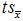# Chapter 6: Estimation and Confidence Intervals

Answers for Data Skill Challenges for every chapter in the book can be found to check your performance and widen your understanding.

1)      Mean = 3.18; s = 1.33; n = 22; df = 21; CI95 = [2.59, 3.77]

2)      Mean = 3.46; σ  = 1.25; n = 8; CI95 = [2.59, 4.33]

3)      Mean = 4.1; s = 0.4; n = 240; df = 239 (but we will use the “200” row, since 239 is not in the t-table);= 1.972 * 0.4/SQRT(240) = 0.050916; ME = 0.050916 / 4.1 = 0.012419 = 1.24%

4)      Mean = 12000; s = 3000; n = 18; df = 17;= 2.110 * 3000/SQRT(18) = 707.106781; ME = 707.106781 / 12000 = 0.058926 = 5.89%